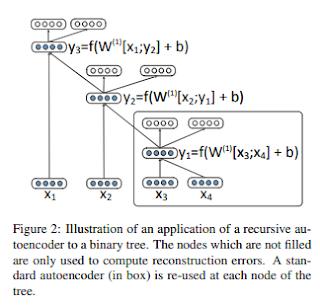## Thursday, March 12, 2020

### Recursive Auto Encoders in Tensorflow 2.0

In my last post, I described recursive neural networks and how they can be used in PyTorch.
In this post, I will describe the recursive autoencoder and my implementation in TensorFlow 2.0 using the new eager execution functionality and the gradient tape.

### What is a recursive autoencoder?Figure from the paper explaining auto encoders

As with the regular autoencoder we build a neural network that decides if we merge two inputs. Instead of having a target, in the autoencoder case we simply reconstruct the input and merge the nodes with the lowest reconstruction error in a greedy manner.

### Implementation

First, we define the auto encoder:

def merge_encoder(n_in):
a = tf.keras.layers.Input(n_in)
b = tf.keras.layers.Input(n_in)
c = tf.keras.layers.Concatenate()([a,b])
h = tf.keras.layers.Dense(n_in)(c)
o = tf.keras.layers.Dense(n_in * 2)(h)
merge = tf.keras.models.Model(inputs=[a, b], outputs=[h, c, o])
return merge

The model has two inputs that should be merged. These will be concatenated and fed through a dense layer resulting in the hidden representation. The hidden representation is directly used to reconstruct the input. In order to keep track of the merges, I define a node class.

class Node:

def __init__(self, i, embedding, score, l = None, r = None):
self.i = i
self.score = score
self.embedding  = embedding
self.left = l
self.right = r

def print(self, offset=""):
print("{} {} {} {}".format(offset, self.i, self.score, np.mean(self.embeding)))
if self.left is not None and self.right is not None:
self.left.print(offset + "\t")
self.right.print(offset + "\t")

def merge(self, other, merger):
merged = merger([self.embedding, other.embedding])
h = merged
c = merged
y = merged
score = tf.nn.l2_loss(y - c) + self.score + other.score
return Node(-1, h, score, self, other)

def ts2leafs(ts):
return [Node(i,ts[i], tf.constant(0.0)) for i in range(0, len(ts))]


A merge holds the embedding, an id for all leaf nodes as well as the merge score. Merging nodes will involve computing the hidden representation for the new node and computing the score from the reconstruction error. At the beginning every vector in a sequence is simply a leaf node. Now we can greedily merge the sequences by finding the minimum possible reconstruction error. We keep merging until only one node is left.


def merge(x, m):
if len(x) > 1:
min_loss = float('inf')
min_node = None
min_i = 0
min_j = 0
for i in range(len(x)):
for j in range(len(x)):
if i < j:
node = x[i].merge(x[j], m)
if node.score < min_loss:
min_node = node
min_loss = node.score
min_i = i
min_j = j
next_x = x.copy()
next_x[min_i] = min_node
next_x = [next_x[idx] for idx in range(0, len(x)) if idx != min_j]
return merge(next_x, m)
else:
return x

Now we can learn the model using TensorFlow's gradient tape which keeps track of all computations during our merges, compute the gradient and update the model.


m = merge_encoder(10)
x = ts2leafs([np.ones((1, 10)) * i for i in range(0, 10)] + [np.ones((1, 10)) * i for i in range(0, 10)])
for i in range(0, 100):